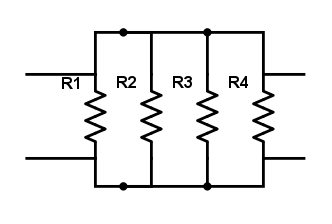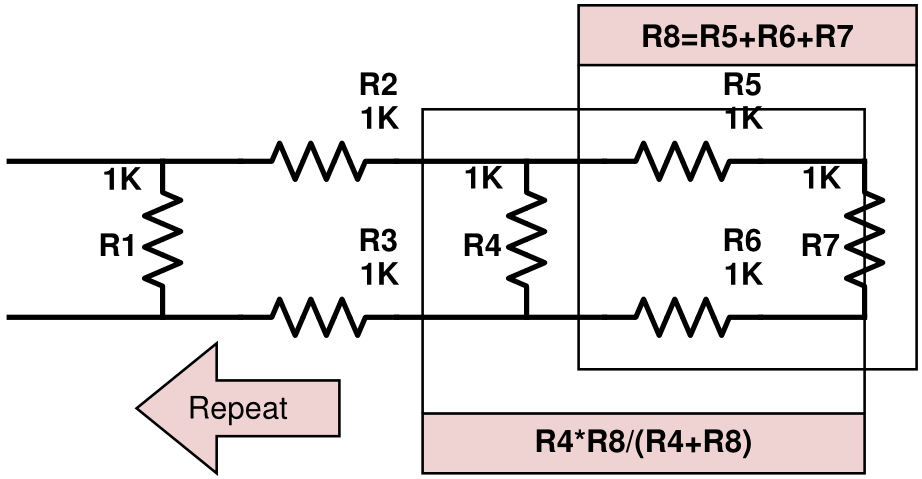This guide to resistors for beginners aims to give you all the knowledge you need for Raspberry Pi and Arduino projects.

One of the most used components is resistors. The main purpose of it is to control the amount of the current that flows through a circuit. Using resistors you can drive the correct power levels on your circuit, and protect other components from a potential damage.

You will be using ‘through-hole’  design, (the one in the main picture) as SMD are tiny (0.8mmx0.5mm) and therefore not practical for a home use. The resistor has 2 pins (or more, if it’s an array) which are bidirectional. The orientation of a resistor on the board does not matter, and there is no polarity of the component.

Resistors can have a variable resistance value, allowing for knobs and sliders to be installed on it, to select the current resistance.

## Schematics

There are 2 ways of marking a resistor in  schematics:both are correct (EU vs USA), and come with labels (R1,R2…etc)  and values  (220Ω, 210kΩ …etc) next to it. There isn’t anything more fascinating to put here really, which is a good thing, as you will notice these schematics get confusing very quickly!

## Bands and power rating

To measure the value of the resistor, and this is something you should do each time if I’m honest,  you can use a multimeter to do so. Additionally, each resistor has colour coded bands (from 4 to 6) that correspond to the value of the resistor.

• Band1 (1st digit)
• Band2 (2nd digit)
• Band3 (if any* 3rd digit)
• Penultimate band (multiplier)
• Last band (tolerance)

Another thing to bear in mind is the power rating of the resistor. Picking lower power rated resistor than required, will result in resistor being fried.  The ones you will come across most often are rated ¼W or ½W.  The rating is measured in Watts.

P= I*V (the formula)
P = I2*R (using Ohm’s Law)
P= V2/R (and if you know the voltage)

## The math & usage

Current LimiterPull-up/Down I/OVoltage divider

### SerialThe math behind the serial link is very simple, just add it all together, to calculate the total resistance of the circuit

Rtot = R1+ R2+R3+R4

This is pretty much all you need to know if you want to know the voltage step downs between the components have a look at the voltage divider tab.

### ParallelThis is where things get little more complicated. While the screen example is relatively simple to deal with, chances are your circuit won’t look that simple.

1/Rtot = 1/R1+1/R2+1/R3+1/R4

This formula allows you to deal with the parallel circuit, there is another version of this calculation, in a slightly more useful shape, and it applies to any 2 resistors in parallel circuit:

Rtot = R1*R2/(R1+R2)

Why am I littering your head with that? Take a look at this:The total resistance value of R8 is calculated like in a serial circuit: the sum of R5,R6,R7, this will give us the base, to use the previous equation for calculating the resistance of 2 resistors in a parallel circuit R8 and R4. This is how we solve more complex situations. From there just rinse and repeat until you get through the entire board. I really hope this makes sense to you!

This part is mostly useful for circuits alone and pull-up behavior is built-in in a lot of microcontrollers (RPI and Arduino). This means that we can enable this within the software. Why should you be interested?  We can set pins to LOW or HIGH,  depending on voltage submitted to the pin. LOW being GND (0V) and HIGH (5V, 3.3V).
There is also another state on input pins. And it is referred to, as floating. This is when the pin is disconnected from the circuit, and can still have a small floating current present (caused by electrical interference), which can cause our circuit to misbehave. Usually, it causes a board to read the pin state as LOW or HIGH regardless of what is happening in our circuit.  To fix this software wise, when declaring your input pin, we can set it as:

``pinMode(5, INPUT_PULLUP);``

which will take care of this issue for us.
If we have no option to do so, we can design a circuit in a way to enable the same functionality.Both circuits deal with the same issue, removing floating electrons on input pins. In the pull-down, when the switch is open – input pin reads LOW, as any floating current is drawn to GND, in a pull-up the logic is inverted, and when the switch is open the input pin reads HIGH as there is a direct connection between the input pin and the power source.

U1, U2 are controllers with pins, which have impedance built-in into the pins itself.  This means that value of our R1 and R2 has to be adjusted accordingly to achieve the pull-up or pull down effect.

Using two resistors in a serial connection we can create a simple voltage divider.Why are we interested?  Unless you are lazy and get yourself bunch of LLC converters, which pretty much plug and play, this basic circuit is a solution to: “I have 5V board and I’m using 3.3V sensor“.  Pretty much any case when Arduino stuff is used on RPI and another way around. It allows you to take the initial Voltage from a power source and step it down to desired value.  We can calculate the V providing we know the values of resistors R1 and R2 and the initial voltage from the power source.

Vout = Vin * [R1/(R1+R2)]

This is used to create a range of potentiometers,  the same principle is used in sensors (photo-sensor: R1 -resistor, R2 Photoresistive component), please don’t use this to lower the power supply voltage (ie stepping down 12V to 6V), as this is very inefficient and can cause problems from overheating, use voltage regulators instead.

I believe this is all you need to know to start working with resistors, whether on its own, or with Raspberry PI and Arduino. I hope that this resistor for beginners guide helped you!

SHARE